Arrays, Tables and Matrices

One of the biggest differences between WebTeX and LaTeX is how arrays are handled. The WebTeX arrays are much more closely related to MathML and HTML tables. In practical terms, this mostly affects the way in which array layout options can be changed, and the command syntax for doing so. At the same time, LaTeX users will also find many familiar features in WebTeX arrays.

Creating an array

The \array command may be used for creating a table of any kind. A simple 2x2 array would be given by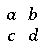\array{ a & b \\
c & d }

An ampersand (&) is placed between entries (called cells) in each row, while rows are separated by a double backslash (\\). Note that there is no & at the end of each row and no \\ after the final row. Spaces and line feeds are ignored by WebEQ; they are only used here to make the WebTeX code easier to read. The expression \array{a&b\\c&d} is displayed the same way as the example above.

Arrays may be nested, as in the example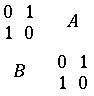\array{ \array{0&1 \\ 1&0} & A \\
B & \array{0&1 \\ 1&0}}


Options

Array options

The first element of an array may be the command \arrayopts{}. This command allows you some control over the layout of the matrix. You may place one or more of the following commands within the braces of \arrayopts{}:

Alignment of columns

Each column may be individually left justified, right justified, or centered. The \colalign command justifies each column according to the list of positions given in its argument. Type one of left, center, or right for each column, separated by spaces. The default is \colalign{center}.

Example: An array with three columns, the first flush left and the other two flush right, is given by

\array{ \arrayopts{\colalign{left right right}} a & b & c}

If there are more columns in the array than there are keywords in \colalign, then all subsequent columns follow the alignment specified by the last keyword. Thus,
\array{ \arrayopts{\colalign{left}} a & b & c}

will align all three columns on the left.

The WebTeX Examples include an example of the alignment of columns within an array.

Alignment of rows

The entries in each row may be collectively raised or lowered with the \rowalign command. As in the alignment of columns mentioned above, rows are adjusted according to the list of positions given in the argument. Type one of top, center, bottom, axis, or baseline for each row, separated by spaces. The default is \rowalign{baseline}.

Example: An array with three rows, the elements of the first row pushed to the top of the row and the elements of the other two pushed to the bottom, is given by

\array{ \arrayopts{\rowalign{top bottom bottom}} a \\ b \\ c}

If there are more rows in the array than there are keywords in \rowalign, then all subsequent rows follow the alignment specified by the last keyword. Thus,
\array{ \arrayopts{\rowalign{top}} a \\ b \\ c}

will place the entry in each row flush with the top of its row.

The WebTeX Examples include an example of the alignment of rows within an array.

Vertical alignment of the whole array

The whole array may be shifted vertically using the \align{} option. The default is \align{center}, which places the center of the array even with the middle of the surrounding expression. Replace center with top or bottom to align the top row or the bottom row with the axis of the surrounding text. A fourth possibility is to align thearray at a certain row. Type \align{r2} to align at the second row.

The WebTeX Examples include an example of the vertical alignment of whole arrays.

WebEQ inserts a small amount of space between entries in an array. The \padding{} command adjusts these gaps. The argument may be any floating point number, including negative numbers. The default is \padding{1}. An argument greater than one spreads the cells, and a number less than one pushes them closer together. Negative values may cause the array entries to overlap.

The WebTeX Examples include an example which shows the effect of using different values for paddings.

Equal-sized rows and columns

The command \equalrows{true} causes all the rows of an array to be the same height. The corresponding command \equalcols{true} does the same for the width of all columns. When the value for one of these options is false, each row or column will be individually scaled to acommodate the entry with the largest height or width. The default for these options is false.

The WebTeX Examples include an example of the use of \equalrows and \equalcols.

Lines between rows and columns

Lines can be drawn between the rows of an array with the command \rowlines. This command has as its argument a list consisting of the keywords solid, dashed, or none, which indicate the type of line used. The lines are specified starting with the line immediately below the top row, and continue to the line above the last row. If there are more gaps between the rows than are specified by keywords, the last keyword is used for the remaining lines.

The insertion of vertical liens between columns is similarly accomplished with the \collines command.

The WebTeX Examples include an example of the use of \rowlines and \collines.

Placing a frame around the array

A box may be drawn around the array with the command \frame. There is one argument, which describes the type of line used. The choices are solid dashed, or none.

Row options

The first element of each row of an array may be the command \rowopts{}. This command allows you some control over the alignment of the text in each row and provides a way to insert horizontal lines between rows.

Alignment of rows

As in array option \rowalign, placing this option within the command \rowopts{} controls the vertical position of each entry in that row. The whole row is aligned vertically according to the option given. The choices are top (the top of each entry is placed flush with the top of the row), bottom (the bottom of each entry sits on the bottom of the row), center (all entries are centered), baseline (all entries align on their natural baselines), and axis. The default is \rowopts{\rowalign{baseline}}, in which the baseline of all entries are matched.

The WebTeX Examples include an example of the alignment of rows.

Horizontal alignment of row entries

The \colalign command has the same format and does the same things when used as an argument of \rowopts as it does when used as an argument of \arrayopts.

Cell options

The command \cellopts{} may be placed at the beginning of any cell with any combination of the following options to adjust that individual cell.

Cell entries covering more than one row or column

These two options, \rowspan and \colspan, allow an entry in the matrix to cover two or more cells. For example, \colspan{2} could be used to create a heading for a table which is centered over the two columns.

The argument of \rowspan is the number of rows including and below the current row that will be occupied by the cell. Subsequent rows affected by the \rowspan cell will have one fewer column, since that cell will take up the column in those rows. Note that there is only one ampersand in the second row of the following example, because the integral will automatically cover the second column in the second row.

\array{ (1,1) & \cellopts{\rowspan{2}} \int dx & (1,3) \\
(2,1) &                                  (2,3) \\
(3,1) & (3,2)                          & (3,3) }


Similarly, the argument of \colspan is the number of columns including and to the right of the current column that will be occupied by the cell. In the following example, the first row contains a cell spanning the first three columns, followed by a cell in the fourth column.

\array{\cellopts{\colspan{3}} (1,1) \text{ to } (1,3) & (1,4) \\
(2,1)  &   (2,2)   &   (2,3)    & (2,4)  }


The WebTeX Examples include further examples of the use of \rowspan and \colspan.

Vertical and horizontal alignment within cells

The cell options \rowalign and \colalign change the vertical or horizontal placement of an entry within its cell, much the same as in array options and the row options, which work on whole columns or rows.

The \rowalign command changes the vertical alignment. The expression in the current cell is placed at the top, center, axis, baseline or bottom of the row by using one of these words as the argument of \rowalign. The default is \rowalign{baseline}, which places the cell entry on the baseline.

Horizontal alignment is controlled by the \colalign command, which has the possible arguments left, center, or right. The default is \colalign{center}.

The WebTeX Examples include an example of the alignment of array entries.

Example: A Matrix

We can take our array example above and turn it into a matrix equation: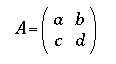A = \left ( \array{ a & b \\
c & d }
\right )

By default, delimiters grow symmetrically around the horizontal axis of the equation. When enclosing a matrix that is aligned at its top or bottom, for example, one needs the delimiters to "float" to match the alignment of the enclosed expression. To accomplish this, use the \floatleft and \floatrightcommands instead.

This example of a 3x2 matrix with its top row aligned with the equals sign requires the use of \floatleft and \floatright.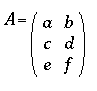A = \floatleft ( \array{ \arrayopts{\align{top}}
a & b \\
c & d \\
e & f }
\floatright )



Example: A function with multiple cases

Another use of WebTeX arrays besides matrices is the creation of a function defined on two or more domains. Here we use a 2x3 array to define the absolute value function: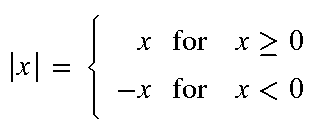\left| x \right| = \left \{
\array{ \arrayopts{\colalign{right center left}
\equalcols{false}}
x & \text{ for } & x \geq 0 \\
-x & \text{ for } & x \lt 0 }
\right


The x's in the first and third columns are lined up using the \collayout array option. The \text commands in the center column ensure that the word for' is in a roman font. Also notice that there is no right delimiter, even though the \right command is required.

The WebTeX Examples include several more examples of the use of arrays.

Example: Commutative diagrams (Overlap commands)

Arrays can also be used to create commutative diagrams. Some overlap commands may be needed to help set the function names at the correct location relative to the arrows. These commands are \llap{} (Argument is left of center), \rlap{} (Argument is right of center), \ulap{} (Argument is above center), and \dlap{} (Argument is below center).

A letter g may be placed above an arrow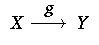X \overset{\ulap{g}}{\longrightarrow} Y


\overset puts the letter above the arrow. Using \ulap gives the effect that the center of the g / arrow combination is aligned with the arrow itself. If we did not use it, the whole structure would be centered, leaving the arrow too low.

To create a square commutative diagram, we use this as the first row of a 3x3 array, placing a & between the letters and the arrow.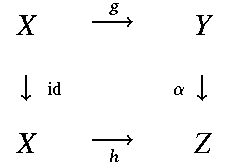\array{ \arrayopts{\rowalign{center axis center}}
X & \overset{\ulap{g}}{\longrightarrow} & Y \\
\downarrow \rlap{\scriptsize{\mathop{id}}} & &
\llap{\scriptsize{\alpha}}{\downarrow} \\
X & \underset{\dlap{h}}{\longrightarrow} & Z
}
`

The WebTeX Examples include an example of a commutative diagram.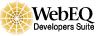Created: Dec 18 1997 --- Last modified: Sat Mar 24 17:25:08 2001